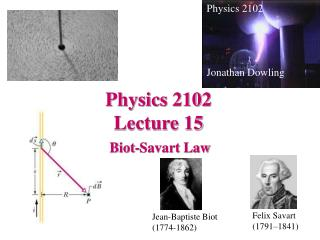Download PresentationPhysics 2102 Lecture 15

# Physics 2102 Lecture 15

Download Presentation## Physics 2102 Lecture 15

- - - - - - - - - - - - - - - - - - - - - - - - - - - E N D - - - - - - - - - - - - - - - - - - - - - - - - - - -
##### Presentation Transcript

1. Physics 2102 Jonathan Dowling Physics 2102 Lecture 15 Biot-Savart Law Felix Savart (1791–1841) Jean-BaptisteBiot (1774-1862)

2. What Are We Going to Learn?A Road Map • Electric charge Electric force on other electric charges Electric field, and electric potential • Moving electric charges : current • Electronic circuit components: batteries, resistors, capacitors • Electric currentsMagnetic field  Magnetic force on moving charges • Time-varying magnetic field  Electric Field • More circuit components: inductors. • Electromagneticwaveslight waves • Geometrical Optics (light rays). • Physical optics (light waves)

3. I Electric Current: A Source of Magnetic Field • Observation: an electric current creates a magnetic field • Simple experiment: hold a current-carrying wire near a compass needle! B Wire with current INTO page B

4. B i i Direction of B??? Yet Another Right Hand Rule! • Point your thumb along the direction of the current in a straight wire • The magnetic field created by the current consists of circular loops directed along your curled fingers. • The magnetic field gets weaker with distance. • You can apply this to ANY straight wire (even a small differential element!) • What if you have a curved wire? Break into small elements.

5. B Superposition OUT OUT • Magnetic fields (like electric fields) can be “superimposed” -- just do a vector sum of B from different sources • The figure shows four wires located at the 4 corners of a square. They carry equal currents in directions indicated • What is the direction of B at the center of the square? IN IN

6. Which we write as, Biot-Savart Law When we computed the electric field due to charges we used Coulomb’s law. If one had a large irregular object, one broke it into infinitesimal pieces and computed, If you wish to compute the magnetic field due to a current in a wire, you use the law of Biot and Savart.

7. The Biot-Savart Law Jean-Baptiste Biot (1774-1862) Felix Savart (1791-1841) i • Quantitative rule for computing the magnetic field from any electric current • Choose a differential element of wire of length dLand carrying a current i • The field dB from this element at a point located by the vector r is given by the Biot-Savart Law m0 =4px10-7 Tm/A (permeability constant)

8. Biot-Savart Law • An infinitely long straight wire carries a current i. • Determine the magnetic field generated at a point located at a perpendicular distance R from the wire. • Choose an element ds as shown • Biot-Savart Law: dB points INTO the page • Integrate over all such elements

9. Field of a Straight Wire

10. Example : A Practical Matter A power line carries a current of 500 A. What is the magnetic field in a house located 100 m away from the power line? = 1 mT!! Recall that the earth’s magnetic field is ~10–4T = 100 mT

11. Biot-Savart Law i • A circular arc of wire of radius R carries a current i. • What is the magnetic field at the center of the loop? Direction of B?? Not another right hand rule?! TWO right hand rules!: If your thumb points along the CURRENT, your fingers will point in the same direction as the FIELD. If you curl our fingers around direction of CURRENT, your thumb points along FIELD!

12. Magnetic field due to wire 1 where the wire 2 is, I1 L I2 Force on wire 2 due to this field, F a Forces between wires

13. Summary • Magnetic fields exert forces on moving charges: • The force is perpendicular to the field and the velocity. • A current loop is a magnetic dipole moment. • Uniform magnetic fields exert torques on dipole moments. • Electric currents produce magnetic fields: • To compute magnetic fields produced by currents, use Biot-Savart’s law for each element of current, and then integrate. • Straight currents produce circular magnetic field lines, with amplitude B=m0i/2pr (use right hand rule for direction). • Circular currents produce a magnetic field at the center (given by another right hand rule) equal to B=m0iF/4pr • Wires currying currents produce forces on each other: parallel currents attract, antiparallel currents repel.